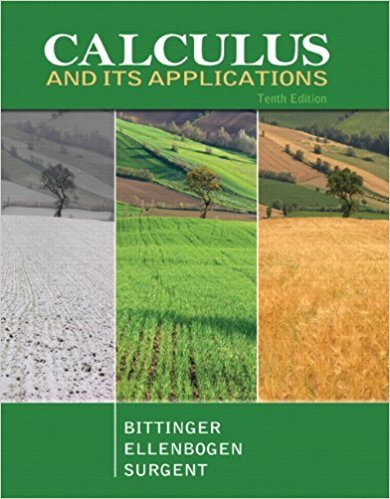×
×

# Solutions for Chapter 4.7: Integration Techniques:Tables## Full solutions for Calculus and Its Applications | 10th Edition

ISBN: 9780321694331Solutions for Chapter 4.7: Integration Techniques:Tables

Solutions for Chapter 4.7
4 5 0 279 Reviews
15
2
##### ISBN: 9780321694331

This textbook survival guide was created for the textbook: Calculus and Its Applications, edition: 10. This expansive textbook survival guide covers the following chapters and their solutions. Calculus and Its Applications was written by and is associated to the ISBN: 9780321694331. Chapter 4.7: Integration Techniques:Tables includes 38 full step-by-step solutions. Since 38 problems in chapter 4.7: Integration Techniques:Tables have been answered, more than 24312 students have viewed full step-by-step solutions from this chapter.

Key Calculus Terms and definitions covered in this textbook
• Additive identity for the complex numbers

0 + 0i is the complex number zero

• Arc length formula

The length of an arc in a circle of radius r intercepted by a central angle of u radians is s = r u.

• Associative properties

a + (b + c) = (a + b) + c, a(bc) = (ab)c.

• Complex fraction

See Compound fraction.

• Cone

See Right circular cone.

• Cotangent

The function y = cot x

• Divisor of a polynomial

See Division algorithm for polynomials.

• Grapher or graphing utility

Graphing calculator or a computer with graphing software.

• Identity matrix

A square matrix with 1’s in the main diagonal and 0’s elsewhere, p. 534.

• Inverse cosine function

The function y = cos-1 x

• Lemniscate

A graph of a polar equation of the form r2 = a2 sin 2u or r 2 = a2 cos 2u.

• Magnitude of a real number

See Absolute value of a real number

• Observational study

A process for gathering data from a subset of a population through current or past observations. This differs from an experiment in that no treatment is imposed.

The distance from a point on a circle (or a sphere) to the center of the circle (or the sphere).

• Relevant domain

The portion of the domain applicable to the situation being modeled.

• Rigid transformation

A transformation that leaves the basic shape of a graph unchanged.

• Sine

The function y = sin x.

• Standard form of a polar equation of a conic

r = ke 1 e cos ? or r = ke 1 e sin ? ,

• Stem

The initial digit or digits of a number in a stemplot.

• Weighted mean

A mean calculated in such a way that some elements of the data set have higher weights (that is, are counted more strongly in determining the mean) than others.

×